# Math in Focus Grade 2 Chapter 16 Practice 3 Answer Key Real-World Problems: Measurement and Money

This handy Math in Focus Grade 2 Workbook Answer Key Chapter 16 Practice 3 Real-World Problems: Measurement and Money detailed solutions for the textbook questions.

## Math in Focus Grade 2 Chapter 16 Practice 3 Answer Key Real-World Problems: Measurement and Money

Tell whether you need to multiply or divide. Then solve. Use bar models to help you.

Example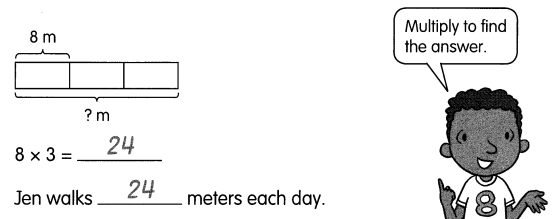Question 1.
Raul cuts 6 strips of paper. Each strip is 5 centimeters long. He tapes them together to make a long strip. How long is the strip he makes?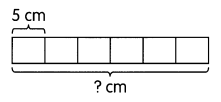5 × 6 = ___________
The strip is ____________ centimeters long.
5 x 6 = 30
The strip is 30 centimeters long.

Tell whether you need to multiply or divide. Then solve. Use bar models to help you.

Question 2.
Helen has a ribbon that is 21 inches long. She cuts it into 3 equal pieces. How long is each piece?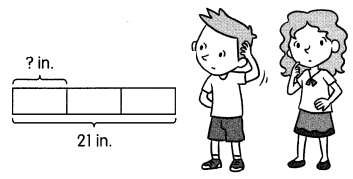21 ÷ 3 = ____________
Each piece is __________ inches long.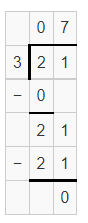21 ÷ 3 = 7
Each piece is 7 inches long.

Question 3.
Jessica has a string that is 30 feet long. She cuts it into equal pieces. Each piece is 5 feet long. How many pieces does she cut the string into?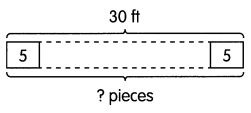She cuts the string into __________ pieces.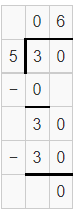30 ÷ 5 = 6
She cuts the string into 6 pieces.

Tell whether you need to multiply or divide. Then solve. Draw bar models to help you.

Question 4.
A strip of paper is 40 centimeters long. It is cut into 4 equal pieces. How long is each piece?
Each piece is __________ centimeters long.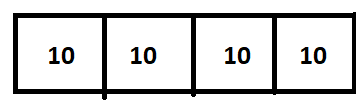40 ÷ 4 = 10
Each piece is 10 centimeters long.

Question 5.
Sara is making curtains. She needs 5 meters of cloth for each curtain. She has 45 meters of cloth. How many curtains can she make?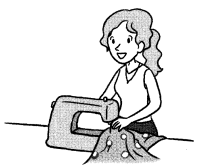She can make __________ curtains.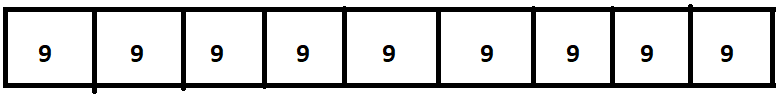45 ÷ 5 = 9
Therefore, she can make 9 curtains.

Tell whether you need to multiply or divide. Then solve. Draw bar models to help you.

Question 6.
The mass of 5 bags of potatoes is 30 kilograms. Each bag has the same mass. What is the mass of each bag?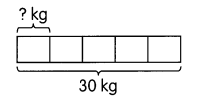The mass of each bag is __________ kilograms.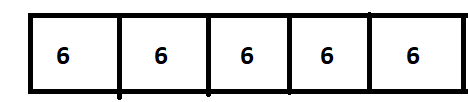30 ÷ 5 = 6
Therefore, the mass of each bag is 6 kilograms.

Question 7.
There are 6 bricks. Each brick has a mass of 3 kilograms. What is the total mass of the 6 bricks?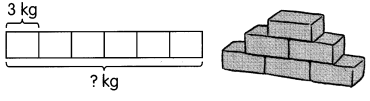The total mass of the 6 bricks is __________ kilograms.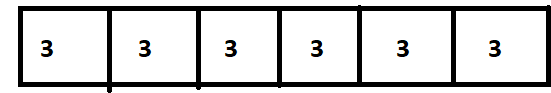6 x 3 = 18
Therefore, the total mass of 6 bricks is 18 kilograms.

Tell whether you need to multiply or divide. Then solve. Draw bar models to help you.

Question 8.
A pencil sharpener has a mass of 10 grams. What is the total mass of 7 pencil sharpeners?
The total mass of 7 pencil sharpeners is __________ grams.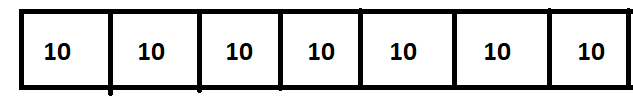So, 10 x 7 = 70
Therefore, The total mass of 7 pencil sharpeners is 10 grams.

Question 9.
The total mass of 3 bags of flour is 6 kilograms. Each bag has the same mass. What is the mass of each bag of flour?
The mass of each bag of flour is __________ kilograms.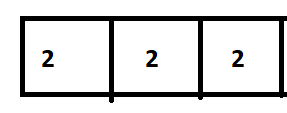So, 6 ÷ 3 = 2
Therefore, The mass of each bag of flour is 2 kilograms.

Tell whether you need to multiply or divide. Then solve. Draw bar models to help you.

Question 10.
32 kilograms of rice is divided equally into some bags. Each bag has a mass of 8 kilograms. How many bags of rice are there?
There are __________ bags of rice.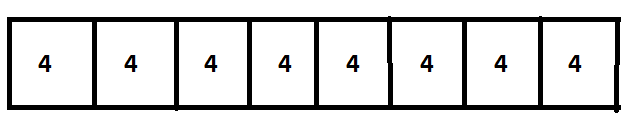S0, 32 ÷ 8 = 4
Therefore, there are 4 bags of rice.

Question 11.
Mrs. Evan’s family drinks 5 liters of milk in a week. How many liters of milk does her family drink in 7 weeks?
Her family drinks __________ liters of milk in 7 weeks.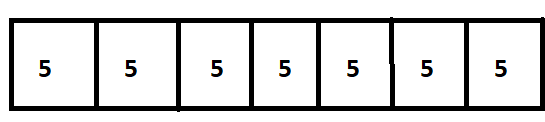So, 5 x 7 =35
Therefore, 35 liters of milk her family drinks in 7 weeks.

Tell whether you need to multiply or divide. Then solve. Draw bar models to help you.

Question 12.
Alberto pours 18 liters of water equally into 3 tanks. How much water is there in each tank?
There are __________ liters of water in each tank.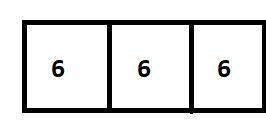So, 18 ÷ 3 = 6
Therefore, 6 liters of water there in each tank.

Question 13.
Barry collects 16 liters of water from a well. He pours the water into some pails. Each pail contains 4- liters of water. How many pails are there in all?
There are __________ pails in all.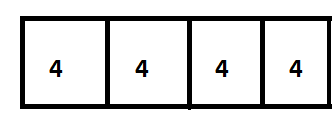So, 16 ÷ 4 = 4
Therefore, there are 4 pails in all.

Tell whether you need to multiply or divide. Then solve. Draw bar models to help you.

Question 14.
Mariam gives $40 to her grandchildren. Each of them gets$5. How many grandchildren does she have?
She has __________ grandchildren.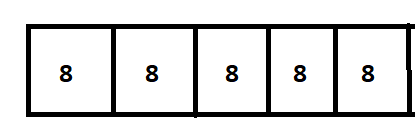Mrs. Tan buys 4 bottles of sauce. Each bottle costs $4. How much does she pay? She pays$ __________.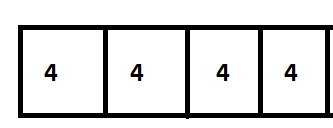Therefore, she paid $16. Question 16. Linda has$50. She spends all of it on some bags. Each bag costs $10. How many bags are there in all? There are __________ bags in all. Answer: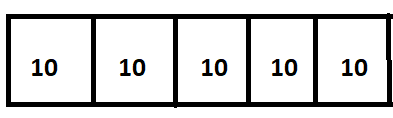So,$50 ÷ \$10 = 5